• 本文会讨论决策树中的分类树与回归树，后续文章会继续讨论决策树的Boosting和Bagging的相关方法。决策树由结点和有向边组成。结点有两种类型：内部结点和叶结点，其中内部结点表示一个特征或属性，叶结点表示一个类...
分类目录：《深入理解机器学习》总目录 相关文章： 基于决策树的模型（一）分类树和回归树 基于树的模型（二）：集成学习之Bagging和Random Forest 基于树的模型（三）：集成学习之GBDT和XGBoost 基于树的模型（四）：随机森林的延伸——深度森林（gcForest） 基于树的模型（五）：从零开始用Python实现ID3决策树 基于树的模型（六）：Python实现CART决策树并利用Tkinter构建GUI对决策树进行调优 基于树的模型（七）：RF/XGBoost等算法实践与决策树Scala实践等（材料准备中）

决策树（Decision Tree）是一种基本的分类与回归方法，当决策树用于分类时称为分类树，用于回归时称为回归树。本文主要讨论决策树中的分类树与回归树的一些基本理论，后续文章会继续讨论决策树的Boosting和Bagging相关方法。
决策树由结点和有向边组成。结点有两种类型：内部结点和叶结点，其中内部结点表示一个特征或属性，叶结点表示一个类。一般的，一棵决策树包含一个根结点、若干个内部结点和若干个叶结点。叶结点对应于决策结果，其他每个结点则对应于一个属性测试。每个结点包含的样本集合根据属性测试的结果被划分到子结点中，根结点包含样本全集，从根结点到每个叶结点的路径对应了一个判定测试序列。在下图中，圆和方框分别表示内部结点和叶结点。决策树学习的目的是为了产生一棵泛化能力强，即处理未见示例能力强的决策树。分类树
分类树是一种描述对实例进行分类的树形结构。在使用分类树进行分类时，从根结点开始，对实例的某一特征进行测试，根据测试结果，将实例分配到其子结点。这时，每一个子结点对应着该特征的一个取值。如此递归地对实例进行测试并分配，直至达到叶结点。最后将实例分到叶结点的类中。
假设给定训练数据集：

D

=

{

(

x

1

,

y

1

)

,

(

x

2

,

y

2

)

,

.

.

.

,

(

x

N

,

y

N

)

}

D=\{(x_1, y_1), (x_2, y_2), ..., (x_N, y_N)\}

其中，

x

i

=

(

x

i

(

1

)

,

x

i

(

2

)

,

.

.

.

,

x

i

(

n

)

)

T

,

x_i=(x_i^{(1)}, x_i^{(2)}, ..., x_i^{(n)})^T,

为输入实例，即特征向量，

n

n

为特征个数，

i

=

1

，

2

…

，

N

i=1，2…，N

，

N

N

为样本容量，

y

i

∈

{

1

,

2

,

.

.

.

,

K

}

y_i \in \{ 1, 2, ..., K\}

为类标。分类树学习的目标是根据给定的训练数据集构建一个决策树模型，使它能够对实例进行正确的分类。
决策树学习本质上是从训练数据集中归纳出一组分类规则。与训练数据集不相矛盾的决策树（即能对训练数据进行正确分类的决策树）可能有多个，也可能一个也没有。我们需要的是一个与训练数据矛盾较小的决策树，同时具有很好的泛化能力。从另一个角度看，决策树学习是由训练数据集估计条件概率模型。基于特征空间划分的类的条件概率模型有无穷多个，我们选择的条件概率模型应该不仅对训练数据有很好的拟合，而且对未知数据有很好的预测。
决策树学习用损失函数表示这一目标，其损失函数通常是正则化的极大似然函数，决策树学习的策略是以损失函数为目标函数的最小化。当损失函数确定以后，学习问题就变为在损失函数意义下选择最优决策树的问题。因为从所有可能的决策树中选取最优决策树是NP完全问题，所以现实中决策树学习算法通常采用启发式方法，近似求解这一最优化问题。这样得到的决策树是次最优的。

决策树分类算法 输入：

训练集：

D

=

(

x

1

,

y

1

)

,

(

x

2

,

y

2

)

,

⋯

,

(

x

N

,

y

N

)

D = {(x_1, y_1), (x_2, y_2), \cdots, (x_N, y_N)}

属性集：

A

=

a

1

,

a

2

,

⋯

,

a

n

A = {a_1, a_2, \cdots, a_n}

过程：

函数

T

r

e

e

G

e

n

e

r

a

t

e

(

D

,

A

)

TreeGenerate(D, A)

输出：

以node为根节点的决策树 算法： （ 1 ） 生成结点根node （ 2 ） if

D

D

中样本全属于同一类别

C

k

C_k

then （ 3 ）

将node标记为

C

k

C_k

类叶结点 （ 4 ）

return （ 5 ） end if （ 6 ） if

A

=

∅

A = \varnothing

OR

D

D

中样本在

A

A

上取值相同 then （ 7 ）

将node标记为叶结点，其类别标记为

D

D

中样本数最多的类 （ 8 ）

return （ 9 ）end if （10）从

A

A

中选择最优划分属性

a

∗

a_*

（11）for

a

∗

a_*

的每一个值

a

∗

v

a_*^v

do （12）

为node生成一个分支：令

D

v

D_v

表示

D

D

中在

a

∗

a_*

上取值为

a

∗

v

a_*^v

的样本子集 （13）

if

D

v

D_v

为空 then （14）

将分支结点标记为叶结点，其类别标记为

D

D

中样本最多的类 （15）

return （16）

else （17）

以

T

r

e

e

G

e

n

e

r

a

t

e

(

D

v

,

A

−

{

a

∗

}

)

TreeGenerate(D_v, A - \{a_*\})

为分支结点 （18）

end if （19）end for

决策树学习的算法通常是一个递归地选择最优特征，并根据该特征对训练数据进行分割，使得对各个子数据集有一个最好的分类的过程。这一过程对应着对特征空间的划分，也对应着决策树的构建。开始，构建根结点，将所有训练数据都放在根结点。选择一个最优特征，按照这一特征将训练数据集分割成子集，使得各个子集有一个在当前条件下最好的分类。如果这些子集已经能够被基本正确分类，那么构建叶结点，并将这些子集分到所对应的叶结点中去，如果还有子集不能被基本正确分类，那么就对这些子集选择新的最优特征，继续对其进行分割，构建相应的结点。如此递归地进行下去，直至所有训练数据子集被基本正确分类，或者没有合适的特征为止。最后每个子集都被分到叶结点上，即都有了明确的类。这就生成了一棵决策树。
从上述过程中就可以看出，决策树的生成是一个递归过程。在决策树基本算法中，有三种情形会导致递归返回
当前结点包含的样本全属于同一类别，无需划分当前属性集为空，或是所有样本在所有属性上取值相同，无法划分当前结点包含的样本集合为空，不能划分
在第二种情形下，我们把当前结点标记为叶结点，并将其类别设定为该结点所含样本最多的类别。在第三种情形下，同样把当前结点标记为叶结点，但将其类别设定为其父结点所含样本最多的类别。这两种情形的处理实质不同：第二种情况是在利用当前结点的后验分布，而第三种情况则是把父结点的样本分布作为当前结点的先验分布。
以上方法生成的决策树可能对训练数据有很好的分类能力，但对未知的测试数据却未必有很好的分类能力，即可能发生过拟合现象。我们需要对已生成的树自下而上进行剪枝，将树变得更简单，从而使它具有更好的泛化能力。具体地，就是去掉过于细分的叶结点，使其回退到父结点，甚至更高的结点，然后将父结点或更高的结点改为新的叶结点。如果特征数量很多，也可以在决策树学习开始的时候，对特征进行选择，只留下对训练数据有足够分类能力的特征。
可以看出，决策树学习算法包含特征选择、决策树的生成与决策树的剪枝过程。由于决策树表示一个条件概率分布，所以深浅不同的决策树对应着不同复杂度的概率模型。决策树的生成对应于模型的局部选择，决策树的剪枝对应于模型的全局选择。决策树的生成只考虑局部最优，相对地，决策树的剪枝则考虑全局最优。
决策树模型呈树形结构，在分类问题中，表示基于特征对实例进行分类的过程。它可以认为是if-then规则的集合，也可以认为是定义在特征空间与类空间上的条件概率分布。分类树具有良好的可读性与分类速度快的优点。分类树在学习时，利用训练数据，根据损失函数最小化的原则建立分类树模型，在预测时，对新的数据，利用分类树模型进行分类。决策树学习通常包括3个步骤：特征选择、决策树的生成和决策树的修剪。
决策树与if-then规则
可以将决策树看成一个if-then规则的集合：由决策树的根结点到叶结点的每一条路径构建一条规则，路径上内部结点的特征对应着规则的条件，而叶结点的类对应着规则的结论。决策树的路径或其对应的if-then规则集合具有一个重要的性质——互斥并且完备。这就是说，每一个实例都被一条路径或一条规则所覆盖，而且只被一条路径或一条规则所覆盖。这里所谓覆盖是指实例的特征与路径上的特征一致或实例满足规则的条件。
决策树与条件概率分布
决策树还表示给定特征条件下类的条件概率分布。这一条件概率分布定义在特征空间的一个划分上。将特征空间划分为互不相交的单元或区域，并在每个单元定义一个类的概率分布就构成了一个条件概率分布。决策树的一条路径对应于划分中的一个单元。决策树所表示的条件概率分布由各个单元给定条件下类的条件概率分布组成。假设

X

X

为表示特征的随机变量，

Y

Y

为表示类的随机变量，那么这个条件概率分布可以表示为

P

（

Y

∣

X

）

P（Y|X）

。

X

X

取值于给定划分下单元的集合，

Y

Y

取值于类的集合。各叶结点（单元）上的条件概率往往偏向某一个类，即属于某一类的概率较大。决策树分类时将该结点的实例强行分到条件概率大的那一类去。
决策树的优缺点
计算复杂度不高对中间缺失值不敏感解释性强，在解释性方面甚至比线性回归更强与传统的回归和分类方法相比，决策树更接近人的决策模式可以用图形表示，非专业人士也可以轻松理解可以直接处理定性的预测变量而不需创建哑变量决策树的预测准确性一般比回归和分类方法弱，但可以通过用集成学习方法组合大量决策树，显著提升树的预测效果
特征选择
特征选择在于选取对训练数据具有分类能力的特征。这样可以提高决策树学习的效率。如果利用一个特征进行分类的结果与随机分类的结果没有很大差别，则称这个特征是没有分类能力的。经验上扔掉这样的特征对决策树学习的精度影响不大。比如，我们希望构建一棵决策树来根据不同人的各种属性来预测每个人性别，那么对于属性“头发的长度”可能就要比属性“头发的颜色”所能包含的信息更多。因为一般来说，男生的头发要比女生的头发短，所以我们希望“头发的长度”这个属性处于决策树的上部。随着划分过程不断进行，我们希望决策树的分支结点所包含的样本尽可能属于同一类别，即结点的“纯度”（purity）越来越高。
信息增益
为了便于说明信息增益，先给出熵与条件熵的定义。在信息论与概率统计中，熵（entropy）是表示随机变量不确定性的度量。设

X

X

是一个取有限个值的离散随机变量，其概率分布为:

P

(

X

=

x

i

)

=

p

i

,

i

=

1

,

2

,

⋯

,

n

P(X = x_i) = p_i, i = 1, 2, \cdots, n

则随机变量

X

X

的熵定义为：

H

(

X

)

=

−

∑

i

=

1

n

p

i

log

⁡

p

i

H(X) = -\sum_{i = 1}^n p_i \log p_i

在上式中，若

p

i

=

0

p_i = 0

，则定义

p

i

log

⁡

p

i

=

0

p_i \log p_i = 0

。通常，上式中的对数以

2

2

为底或以

e

e

为底（自然对数），这时熵的单位分别称作比特（bit）或纳特（nat）.由定义可知，熵只依赖于

X

X

的分布，而与

X

X

的取值无关，所以也可将

X

X

的熵记作

H

(

p

)

H(p)

，即:

H

(

p

)

=

−

∑

i

=

1

n

p

i

log

⁡

p

i

H(p) = -\sum_{i = 1}^n p_i \log p_i

由此可见，熵越大，随机变量的不确定性就越大。从熵的定义可验证

0

≤

H

(

p

)

≤

log

⁡

n

0 \leq H(p) \leq \log n

当随机变量只取两个值，例如1，0时，即

X

X

的分布为:

P

(

X

=

1

)

=

p

,

P

(

X

=

0

)

=

1

−

p

,

0

≤

p

≤

1

P(X = 1) = p,\quad P(X = 0) = 1-p, \quad 0≤p≤1

其熵为：

H

(

p

)

=

−

p

log

⁡

2

p

−

(

1

−

p

)

log

⁡

2

(

1

−

p

)

H(p) = -p \log_2 p - (1 - p)\log_2 (1 - p)

这时，熵

H

(

p

)

H(p)

随概率

p

p

变化的曲线如下图所示（单位为比特）：当

p

=

0

p = 0

或

p

=

1

p = 1

时

H

(

p

)

=

0

H(p) = 0

，随机变量完全没有不确定，当

p

=

0.5

p = 0.5

时，

H

(

p

)

=

1

H(p) = 1

，熵取值最大，随机变量不确定性最大。
设有随机变量

(

X

,

Y

)

(X, Y)

，其联合概率分布为：

P

(

X

=

x

i

,

Y

=

y

i

)

=

p

i

j

{

i

=

1

,

2

,

⋯

,

n

j

=

1

,

2

,

⋯

,

m

P(X = x_i, Y = y_i) = p_{ij} \quad \begin{cases} i = 1, 2, \cdots, n \\ j = 1, 2, \cdots, m \end{cases}

条件熵

H

(

Y

∣

X

)

H(Y|X)

表示在已知随机变量

X

X

的条件下随机变量

Y

Y

的不确定性。随机变量

X

X

给定的条件下随机变量

Y

Y

的条件熵（conditional entropy）

H

(

Y

∣

X

)

H(Y|X)

，定义为

X

X

给定条件下

Y

Y

的条件概率分布的熵对

X

X

的数学期望：

H

(

Y

∣

X

)

=

∑

i

=

1

n

p

i

H

(

Y

∣

X

=

x

i

)

H(Y|X) = \sum_{i = 1}^n p_iH(Y|X = x_i)

其中，

p

i

=

P

(

X

=

x

i

)

,

i

=

1

,

2

,

⋯

,

n

p_i = P(X = x_i), i = 1, 2, \cdots, n

。
当熵和条件熵中的概率由数据估计（如极大似然估计）得到时，所对应的熵与条件熵分别称为经验熵（empirical entropy）和经验条件熵（empirical conditional entropy）。此时，如果有0概率，令

0

log

⁡

0

=

0

0\log0 = 0

。
信息增益（information gain）表示得知特征

X

X

的信息而使得类

Y

Y

的信息的不确定性减少的程度。特征

a

∗

a_*

对训练数据集

D

D

的信息增益

g

(

D

,

a

∗

)

g(D, a_*)

，定义为集合

D

D

的经验熵

H

(

D

)

H(D)

与特征

a

∗

a_*

给定条件下

D

D

的经验条件熵

H

(

D

∣

a

∗

)

H(D|a_*)

之差，即：

g

(

D

,

a

∗

)

=

H

(

D

)

−

H

(

D

∣

a

∗

)

g(D, a_*) = H(D) - H(D|a_*)

一般地，熵

H

(

Y

)

H(Y)

与条件熵

H

(

Y

∣

X

)

H(Y|X)

之差称为互信息（mutual information）。决策树学习中的信息增益等价于训练数据集中类与特征的互信息。
决策树学习应用信息增益准则选择特征。给定训练数据集

D

D

和特征

a

∗

a_*

，经验熵

H

(

D

)

H(D)

表示对数据集

D

D

进行分类的不确定性。而经验条件熵

H

(

D

∣

a

∗

)

H(D|a_*)

表示在特征

a

∗

a_*

给定的条件下对数据集

D

D

进行分类的不确定性。那么它们的差，即信息增益，就表示由于特征

a

∗

a_*

而使得对数据集

D

D

的分类的不确定性减少的程度。显然，对于数据集

D

D

而言，信息增益依赖于特征，不同的特征往往具有不同的信息增益，信息增益大的特征具有更强的分类能力。
根据信息增益准则的特征选择方法：对训练数据集（或子集）

D

D

，计算其每个特征的信息增益，并比较它们的大小，选择信息增益最大的特征。
设训练数据集为

D

D

，

∣

D

∣

|D|

表示其样本容量，即样本个数。设有

K

K

个类

C

k

C_k

，

k

=

1

,

2

,

⋯

,

K

k=1, 2, \cdots, K

，

∣

C

k

∣

|C_k|

为属于类

C

k

C_k

的样本个数，

∑

k

=

1

K

∣

C

k

∣

=

∣

D

∣

\sum_{k=1}^K |C_k| = |D|

。设特征

a

∗

a_*

有

V

V

个不同的取值

{

a

∗

1

,

a

∗

2

,

⋯

,

a

∗

V

}

\{ a_*^1, a_*^2, \cdots, a_*^V\}

，根据特征

a

∗

a_*

的取值将

D

D

划分为

V

V

个子集

D

1

,

D

2

,

⋯

,

D

V

D_1, D_2, \cdots, D_V

，

∣

D

t

∣

|D_t|

为

D

t

D_t

的样本个数，

∑

i

=

1

n

∣

D

t

∣

=

∣

D

∣

\sum_{i=1}^n|D_t|=|D|

。记子集

D

i

D_i

中属于类

C

k

C_k

的样本的集合为

D

i

k

D_{ik}

。即

D

i

k

=

D

i

∩

C

k

D_{ik} = D_i \cap C_k

，

∣

D

i

k

∣

|D_{ik}|

为

D

i

k

D_{ik}

的样本个数。于是计算信息增益的方法如下：

信息增益 输入：训练数据集

D

D

和特征

a

∗

a_*

输出：特征

a

∗

a_*

对训练数据集

D

D

的信息增益

g

(

D

,

a

∗

)

g(D, a_*)

1.计算数据集

D

D

的经验熵

H

(

D

)

H(D)

：

H

(

D

)

=

−

∑

k

=

1

K

C

k

D

log

⁡

2

C

k

D

H(D) = -\sum_{k=1}^K \frac{C_k}{D}\log_2\frac{C_k}{D}

2.计算特征

A

A

对数据集

D

D

的经验条件熵

H

(

D

∣

A

)

H(D|A)

：

H

(

D

∣

A

)

=

∑

i

=

1

n

∣

D

i

∣

D

H

(

D

i

)

=

−

∑

i

=

1

n

∣

D

i

∣

D

∑

k

=

1

K

D

i

k

D

i

log

⁡

2

D

i

k

D

i

H(D|A) = \sum_{i=1}^n\frac{|D_i|}{D}H(D_i)=-\sum_{i=1}^n\frac{|D_i|}{D}\sum_{k=1}^K\frac{D_{ik}}{D_i}\log_2\frac{D_{ik}}{D_i}

3.计算信息增益：

g

(

D

,

a

∗

)

=

H

(

D

)

−

H

(

D

∣

a

∗

)

g(D, a_*) = H(D) - H(D|a_*)

一般而言，信息增益越大，则意味着使用特征

a

∗

a_*

来进行划分所获得的“纯度提升”越大。因此，我们可用信息增益来进行决策树的划分属性选择，即在上述决策树分类算法第10行使用

a

∗

=

arg

max

⁡

a

∈

A

g

(

D

,

a

)

a_* = \text{arg}\ \max_{a \in A}g(D, a)

选择最优划分属性。著名的ID3决策树学习算法就是以信息增益为准则来选择划分属性。
ID3算法的核心是在决策树各个结点上应用信息增益准则选择特征，递归地构建决策树。具体方法是：从根结点开始，对结点计算所有可能的特征的信息增益，选择信息增益最大的特征作为结点的特征，由该特征的不同取值建立子结点。之后，再对子结点递归地调用以上方法，构建决策树，直到所有特征的信息增益均很小或没有特征可以选择为止，最终得到一个决策树。ID3相当于用极大似然法进行概率模型的选择。

ID3算法 输入：训练数据集

D

D

，特征集

A

A

，阈值

ϵ

\epsilon

输出：决策树

T

T

1.若

D

D

中所有实例属于同一类

C

k

C_k

，则

T

T

为单结点树，并将类

C

k

C_k

作为该结点的类标记，返回决策树

T

T

2.若

A

=

∅

A = \varnothing

，则

T

T

为单结点树，并将

D

D

中实例数最大的类

C

k

C_k

，作为该结点的类标记，返回决策树

T

T

3.否则，计算

A

A

中各特征对

D

D

的信息增益，选择信息增益最大的特征

a

∗

a_*

4.如果

a

∗

a_*

的信息增益小于阈值

ϵ

\epsilon

，则置

T

T

为单结点树，并将

D

D

中实例数最大的类

C

k

C_k

作为该结点的类标记，返回决策树

T

T

5.否则，对

a

∗

a_*

的每一可能值

a

∗

v

a_*^v

，依

a

∗

=

a

∗

v

a_* = a_*^v

将

D

D

分割为若干非空子集

D

v

D_v

，将

D

v

D_v

中实例数最大的类作为标记，构建子结点，由结点及其子结点构成决策树

T

T

，返回决策树

T

T

6.对第

v

v

个子结点，以

D

v

D_v

为训练集，以

A

−

{

a

∗

}

A - \{a_*\}

为特征集，递归地调用第(1)步~第(5)步，得到子树

T

v

T_v

，并返回子树

T

v

T_v

信息增益率
信息增益值的大小是相对于训练数据集而言的，并没有绝对意义。在训练数据集的经验熵大的时候，信息增益值会偏大。反之，信息增益值会偏小。使用信息增益率（information gain ratio）可以对这一问题进行校正。这是特征选择的另一准则。特征

a

∗

a_*

对训练数据集

D

D

的信息增益率

g

g

(

D

,

a

∗

)

g_g(D, a_*)

定义为其信息增益

g

(

D

,

a

∗

)

g(D, a_*)

与训练数据集

D

D

的经验熵

H

(

D

)

H(D)

之比：

g

g

(

D

,

a

∗

)

=

g

(

D

,

a

∗

)

H

(

D

)

g_g(D, a_*) = \frac{g(D, a_*)}{H(D)}

如前文所说，信息增益准则对可取值数目较多的属性有所偏好，为减少这种偏好可能带来的不利影响，著名的C4.5决策树算法不直接使用信息增益来选择划分属性，而是使用信息增益率来选择最优划分属性。

C4.5算法 输入：训练数据集

D

D

，特征集

A

A

，信息增益率阈值

ϵ

\epsilon

，信息增益阈值

α

\alpha

输出：决策树

T

T

1.若

D

D

中所有实例属于同一类

C

k

C_k

，则

T

T

为单结点树，并将类

C

k

C_k

作为该结点的类标记，返回决策树

T

T

2.若

A

=

∅

A = \varnothing

，则

T

T

为单结点树，并将

D

D

中实例数最大的类

C

k

C_k

，作为该结点的类标记，返回决策树

T

T

3.否则，计算

A

A

中各特征对

D

D

的信息增益和信息增益率，在信息增益大于

α

\alpha

的特征中选择信息增益率最大的特征

a

∗

a_*

4.如果

a

∗

a_*

的信息增益率小于阈值

ϵ

\epsilon

，则置

T

T

为单结点树，并将

D

D

中实例数最大的类

C

k

C_k

作为该结点的类标记，返回决策树

T

T

5.否则，对

a

∗

a_*

的每一可能值

a

∗

v

a_*^v

，依

a

∗

=

a

∗

v

a_* = a_*^v

将

D

D

分割为若干非空子集

D

v

D_v

，将

D

v

D_v

中实例数最大的类作为标记，构建子结点，由结点及其子结点构成决策树

T

T

，返回决策树

T

T

6.对第

v

v

个子结点，以

D

v

D_v

为训练集，以

A

−

{

a

∗

}

A - \{a_*\}

为特征集，递归地调用第(1)步~第(5)步，得到子树

T

v

T_v

，并返回子树

T

v

T_v

需注意的是，信息增益率准则对可取值数目较少的属性有所偏好，因此，C4.5算法并不是直接选择增益率最大的候选划分属性，而是使用了一个启发式的方法选择最优划分属性：先从候选划分属性中找出信息增益高于平均水平的属性，再从中选择增益率最高的.。
连续值处理
实际的任务中常会遇到连续属性，对于全部为连续属性的样本来说，我们一般使用回归决策树来处理。C4.5算法则采用了二分法对连续属性进行处理。由于连续属性的可取值数目不再有限，因此，不能直接根据连续属性的可取值来对结点进行划分。此时，连续属性离散化技术可派上用场。最简单的策略是采用二分法对连续属性进行处理。
给定样本集

D

D

和连续属性

a

a

，假定

a

a

在

D

D

上出现了

n

n

个不同的取值，将这些值从小到大进行排序，记为

{

a

1

,

a

2

,

a

3

,

⋯

,

a

n

}

\{a_1, a_2, a_3, \cdots, a_n\}

。基于划分点

t

t

可将

D

D

分为子集

D

t

+

D_t^+

和

D

t

−

D_t^-

，其中

D

t

+

D_t^+

包含那些在属性

a

a

上取值大于

t

t

的样本，而

D

t

−

D_t^-

则包含那些在属性

a

a

上取值不大于

t

t

的样本。显然，对相邻的属性取值

a

i

a^i

与

a

i

+

1

a^{i + 1}

来说，

t

t

在区间

[

a

i

,

a

i

+

1

)

[a^i, a^{i + 1})

中取任意值所产生的划分结果相同.因此，对连续属性

a

a

，我们可考察包含

n

−

1

n-1

个元素的候选划分点集合：

T

a

=

{

a

i

+

a

i

+

1

2

∣

1

≤

i

≤

n

−

1

}

T_a = \{\frac{a^i + a^{i + 1}}{2} \ | \ 1 \leq i \leq n - 1\}

即把区间

[

a

i

,

a

i

+

1

)

[a^i, a^{i + 1})

的中位点

a

i

+

a

i

+

1

2

\frac{a^i + a^{i + 1}}{2}

作为候选划分点。然后，我们就可像离散属性值一样来考察这些划分点，选取最优的划分点进行样本集合的划分：

G

a

i

n

(

D

,

a

)

=

max

⁡

t

∈

T

a

G

a

i

n

(

D

,

a

,

t

)

=

max

⁡

t

∈

T

a

E

n

t

(

D

)

−

∑

λ

∈

{

−

,

+

}

D

t

λ

D

E

n

t

(

D

t

λ

)

\begin{aligned} Gain(D, a) &= \max_{t \in T_a} Gain(D, a, t)\\ & = \max_{t \in T_a} Ent(D) - \sum_{\lambda \in \{-, +\}} \frac{D^\lambda _t}{D}Ent(D^\lambda _t) \end{aligned}

其中

G

a

i

n

(

D

,

a

,

t

)

Gain(D, a, t)

是样本集

D

D

基于划分点

t

t

二分后的信息增益。于是，我们就可选择使

G

a

i

n

(

D

,

a

,

t

)

Gain(D, a, t)

最大化的划分点。
缺失值处理
现实任务中常会遇到不完整样本，即样本的某些属性值缺失。且在属性数目较多的情况下，有时会有大量样本出现缺失值。如果简单地放弃不完整样本，仅使用无缺失值的样本来进行学习，显然是对数据信息极大的浪费。显然，有必要考虑利用有缺失属性值的训练样例来进行学习。
划分属性的选择
给定训练集

D

D

和属性

a

a

，令

D

~

\tilde{D}

表示

D

D

中在属性

a

a

上没有缺失值的样本子集。显然，我们仅可根据

D

~

\tilde{D}

来判断属性

a

a

的优劣。假定属性

a

a

有

V

V

个可取值

{

a

1

,

a

2

,

a

3

,

⋯

,

a

V

}

\{a^1, a^2, a^3, \cdots, a^V\}

，令

D

~

v

\tilde{D}^v

表示

D

~

\tilde{D}

中在属性

a

a

上取值为

a

v

a^v

的样本子集，

D

~

k

\tilde{D}_k

表示

D

~

\tilde{D}

中属于第

k

k

类

(

k

=

1

,

2

,

3

,

⋯

,

K

)

(k = 1, 2, 3, \cdots, K)

的样本子集，则显然有

D

~

=

⋃

k

=

1

K

D

~

k

\tilde{D} = \bigcup^K_{k = 1}\tilde{D}_k

与

D

~

=

⋃

v

=

1

V

D

~

v

\tilde{D} = \bigcup^V_{v = 1}\tilde{D}^v

。假定我们为每个样本

x

x

赋予一个权重

ω

x

\omega_x

，并定义：

ρ

=

∑

x

∈

D

~

ω

x

∑

x

∈

D

ω

x

p

~

k

=

∑

x

∈

D

~

k

ω

x

∑

x

∈

D

~

ω

x

r

~

v

=

∑

x

∈

D

~

v

ω

x

∑

x

∈

D

~

ω

x

\begin{aligned} \rho & = \frac{\sum_{x \in \tilde{D}}\omega_x}{\sum_{x \in D}\omega_x}\\ \tilde{p}_k & = \frac{\sum_{x \in \tilde{D}_k}\omega_x}{\sum_{x \in \tilde{D}}\omega_x}\\ \tilde{r}_v & = \frac{\sum_{x \in \tilde{D}^v}\omega_x}{\sum_{x \in \tilde{D}}\omega_x} \end{aligned}

直观地看，对于属性

a

a

，

ρ

\rho

表示无缺失值样本所占的比例，

p

~

k

\tilde{p}_k

表示无缺失值样本中第

k

k

类所占的比例，

r

~

v

\tilde{r}_v

则表示无缺失值样本中在属性

a

a

上取值

a

v

a^v

的样本所占的比例。基于上述定义，我们可将信息增益的计算式推广为：

G

a

i

n

(

D

,

a

)

=

ρ

×

G

a

i

n

(

D

~

,

a

)

=

ρ

×

(

E

n

t

(

D

~

)

−

∑

x

=

1

V

r

~

v

E

n

t

(

D

~

v

)

)

\begin{aligned} Gain(D, a) &= \rho \times Gain(\tilde{D}, a)\\ & = \rho \times (Ent(\tilde{D}) - \sum_{x = 1}^V \tilde{r}_v Ent(\tilde{D}^v)) \end{aligned}

对样本进行划分
根据上面的定义，若样本

x

x

在划分属性

a

a

上的取值已知，则将

x

x

划入与其取值对应的子结点，且样本权值在子结点中保持为

ω

x

\omega_x

。若样本

x

x

在划分属性

a

a

上的取值未知，则将

x

x

同时划入所有子结点，且样本权值在与属性值

a

v

a^v

对应的子结点中调整为

r

~

v

×

ω

x

\tilde{r}_v \times \omega_x

。直观地看，这就是让同一个样本以不同的概率划入到不同的子结点中去。
基尼指数
数据集

D

D

的纯度还可用基尼值来度量：

G

i

n

i

(

D

)

=

∑

k

=

1

K

p

k

(

1

−

p

k

)

=

1

−

∑

k

=

1

K

p

k

2

Gini(D) = \sum^K_{k=1}p_k(1 - p_k) = 1 - \sum^K_{k=1}p_k^2

其中，

K

K

为类别数，

p

k

p_k

为样本点属于第

k

k

类的概率。对于二类分类问题，若样本点属于第1个类的概率是

p

p

，则概率分布的基尼指数为：

G

i

n

i

(

D

)

=

2

p

(

1

−

p

)

Gini(D) = 2p(1 - p)

直观来说，

G

i

n

i

(

D

)

Gini(D)

反映了从数据集

D

D

中随机抽取两个样本，其类别标记不一致的概率。因此，

G

i

n

i

(

D

)

Gini(D)

越小，则数据集

D

D

的纯度越高。对于属性

a

a

的基尼指数定义为:

G

i

n

i

_

i

n

d

e

x

(

D

,

a

)

=

∑

v

=

1

V

D

v

D

G

i

n

i

(

D

v

)

Gini\_index(D, a) = \sum^V_{v = 1}\frac{D^v}{D}Gini(D^v)

在CART算法中，我们在候选属性集合

A

A

中，选择那个使得划分后基尼指数最小的属性作为最优划分属性，即：

a

∗

=

arg

min

⁡

a

∈

A

G

i

n

i

_

i

n

d

e

x

(

D

,

a

)

a_* = \text{arg}\min_{a \in A}Gini\_index(D, a)

在二类分类问题中，基尼指数

G

i

n

i

(

D

)

Gini(D)

、熵

H

(

p

)

H(p)

的一半，和分类误差率的关系：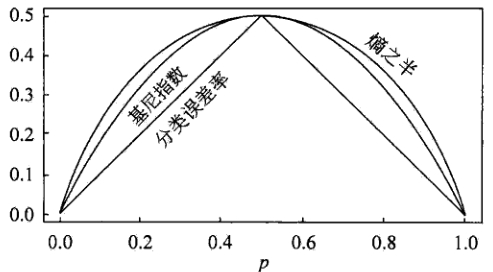其中，横坐标表示概率

p

p

，纵坐标表示损失。可以看出基尼指数和熵的一半的曲线很接近，都可以近似地代表分类误差率。
分类树的剪枝
剪枝（pruning）是决策树学习算法对付“过拟合”的主要手段。在决策树学习中，为了尽可能正确分类训练样本，结点划分过程将不断重复，有时会造成决策树分支过多，这时就可能因为对训练样本学习得“太好”了，以致于把训练集自身的一些特点当作所有数据都具有的一般性质而导致过拟合。因此，可通过主动去掉一些分支来降低过拟合的风险。决策树剪枝的基本策略有预剪枝和后剪枝。
预剪枝
预剪枝是指在决策树生成过程中，对每个结点在划分前先进行估计，若当前结点的划分不能带来决策树泛化性能提升，则停止划分并将当前结点标记为叶结点。停止决策树生长常用方法：
定义一个高度，当决策树达到该高度时就停止决策树的生长达到某个节点的实例具有相同的特征向量，即使这些实例不属于同一类，也可以停止决策树的生长。这个方法对于处理数据的数据冲突问题比较有效。定义一个阈值，当达到某个节点的实例个数小于阈值时就可以停止决策树的生长定义一个阈值，通过计算每次扩张对系统性能的增益，并比较增益值与该阈值大小来决定是否停止决策树的生长。
后剪枝
后剪枝则是先从训练集生成一棵完整的决策树，然后自底向上地对非叶结点进行考察，若将该结点对应的子树替换为叶结点能带来决策树泛化性能提升，则将该子树替换为叶结点。相比于预剪枝，后剪枝更常用，因为在预剪枝中精确地估计何时停止树增长很困难。
错误率降低剪枝（REP，Reduced-Error Pruning）
错误率降低剪枝方法是一种比较简单的后剪枝的方法。在该方法中，可用的数据被分成两个样例集合：首先是训练集，它被用来形成学习到的决策树，另一个是与训练集分离的验证集，它被用来评估这个决策树在后续数据上的精度，确切地说是用来评估修剪这个决策树的影响。学习器可能会被训练集中的随机错误和巧合规律所误导，但验证集合不大可能表现出同样的随机波动。所以验证集可以用来对过度拟合训练集中的虚假特征提供防护检验。
错误率降低剪枝方法考将树上的每个节点作为修剪的候选对象，再决定是对该节点进行剪枝：
删除以此结点为根的子树使其成为叶子结点当修剪后的树对于验证集合的性能不比修剪前的树的性能差时，则确认删除该结点，否则恢复该节点
因为训练集合的过拟合，使得验证集合数据能够对其进行修正，反复进行上面的操作，从底向上的处理结点，删除那些能够提高验证集合的精度的结点，直到进一步修剪会降低验证集合的精度为止。
错误率降低剪枝方法是最简单的后剪枝方法之一，不过由于使用独立的测试集，原始决策树相比，修改后的决策树可能偏向于过度修剪。这是因为一些不会再次在测试集中出现的很稀少的训练集实例所对应的分枝在剪枝过程中往往会被剪枝。尽管错误率降低剪枝方法有这个缺点，不过错误率降低剪枝方法仍然作为一种基准来评价其它剪枝算法的性能。它对于两阶段决策树学习方法的优点和缺点提供了一个很好的学习思路。由于验证集合没有参与决策树的构建，所以用错误率降低剪枝方法剪枝后的决策树对于测试样例的偏差要好很多，能够解决一定程度的过拟合问题。
悲观错误剪枝（PEP，Pesimistic-Error Pruning）
悲观错误剪枝方法是根据剪枝前后的错误率来判定子树的修剪。它不需要像错误率降低修剪方法那样，需要使用部分样本作为测试数据，而是完全使用训练数据来生成决策树，并进行剪枝，即决策树生成和剪枝都使用训练集。
该方法引入了统计学中连续修正的概念弥补错误率降低剪枝方法中的缺陷，在评价子树的训练错误公式中添加了一个常数，即假定每个叶子结点都自动对实例的某个部分进行错误的分类。
把一颗具有多个叶子节点的子树的分类用一个叶子节点来替代的话，在训练集上的误判率肯定是上升的，但是在新数据上不一定。于是我们把子树的误判计算加上一个经验性的惩罚因子来做是否剪枝的考量指标。对于一个叶子节点，它覆盖了

N

N

个样本，其中有

E

E

个错误，那么该叶子节点的错误率为

E

+

0.5

N

\frac{E + 0.5}{N}

。这个

0.5

0.5

就是惩罚因子，那么一颗子树，它有

L

L

个叶子节点，那么该子树的误判率估计为：

∑

E

i

+

0.5

∗

L

∑

N

i

\frac{\sum E_i +0.5 * L}{\sum N_i}

这样的话，我们可以看到一棵子树虽然具有多个子节点，但由于加上了惩罚因子，所以子树的误判率计算未必占到便宜。剪枝后内部节点变成了叶子节点，其误判个数

E

E

也需要加上一个惩罚因子，变成

E

+

0.5

E+0.5

，那么子树是否可以被剪枝就取决于剪枝后的错误

E

+

0.5

E+0.5

在的标准误差内。对于样本的误差率

e

e

，我们可以根据经验把它估计成各种各样的分布模型，比如二项式分布、正态分布等。如果

E

+

0.5

<

E

i

+

S

E

(

E

i

)

E+0.5 < E_i + SE(E_i)

则对

i

i

进行剪枝。
代价复杂度剪枝（CCP，Cost-Complexity Pruning)
代价复杂度剪枝算法为子树

T

t

T_t

定义了代价和复杂度，以及一个可由用户设置的衡量代价与复杂度之间关系的参数

α

α

。其中，代价指在剪枝过程中因子树

T

t

T_t

被叶节点替代而增加的错分样本，复杂度表示剪枝后子树

T

t

T_t

减少的叶结点数，

α

α

则表示剪枝后树的复杂度降低程度与代价间的关系，定义为：

α

=

R

(

t

)

−

R

(

T

t

)

∣

N

t

∣

−

1

\alpha = \frac{R(t) - R(T_t)}{|N_t| - 1}

其中，

∣

N

t

∣

|N_t|

是子树

T

t

T_t

中的叶节点数，

R

(

t

)

=

r

(

t

)

∗

p

(

t

)

R(t) = r(t) * p(t)

为结点

t

t

的错误代价，

r

(

t

)

r(t)

为结点

t

t

的错分样本率，

p

(

t

)

p(t)

为落入结点

t

t

的样本占所有样本的比例，

R

(

T

t

)

=

∑

R

(

i

)

R(T_t) = \sum R(i)

是子树

T

t

T_t

错误代价，

i

i

为子树

T

t

T_t

的叶节点。
对于完全决策树

T

T

的每个非叶结点计算

α

α

值，循环剪掉具有最小

α

α

值的子树，直到剩下根节点，得到一系列的剪枝树

{

T

0

,

T

‘

1

,

T

2

,

⋯

,

T

m

}

\{ T_0, T_1, T_2, \cdots, T_m \}

，其中

T

0

T_0

为原有的完全决策树，

T

m

T_m

为根结点，

T

i

+

1

T_{i +1}

为对

T

i

T_i

进行剪枝的结果从子树序列中，根据真实的误差估计选择最佳决策树
REPPEPCCP剪枝方式自底向上自顶向下自底向上计算复杂度

O

(

n

)

O(n)

O

(

n

)

O(n)

O

(

n

2

)

O(n^2)

误差估计测试集上误差估计使用连续纠正标准误差
回归树
建立回归树的过程大致可以分为两步：
将预测变量空间（

X

1

,

X

2

,

X

3

,

⋯

,

X

p

X_1, X_2, X_3, \cdots, X_p

）的可能取值构成的集合分割成

J

J

个互不重叠的区域

{

R

1

,

R

2

,

R

3

,

⋯

,

R

J

}

\{ R_1, R_2, R_3, \cdots, R_J\}

对落入区域

R

j

R_j

的每个观测值作同样的预测，预测值等于

R

j

R_j

上训练集的各个样本取值的算术平均数。
比如，在第一步中得到两个区域

R

1

R_1

和

R

2

R_2

，

R

1

R_1

中训练集的各个样本取值的算术平均数为10，

R

2

R_2

中训练集的各个样本取值的算术平均数为20。则对给定的观测值

X

=

x

X = x

，若

x

∈

R

1

x \in R_1

，给出的预测值为10，若

x

∈

R

2

x \in R_2

，则预测值为20。
所以，类似于上述决策树分类算法的第(10)步，关键在于如何构建区域划分

{

R

1

,

R

2

,

R

3

,

⋯

,

R

J

}

\{ R_1, R_2, R_3, \cdots, R_J\}

。事实上，区域的形状是可以为任意形状的，但出于模型简化和增强可解释性的考虑，这里将预测变量空间划分成高维矩形，我们称这些区域为称盒子。划分区域的目标是找到使模型的残差平方和

R

S

S

最小的矩形区域

{

R

1

,

R

2

,

R

3

,

⋯

,

R

J

}

\{ R_1, R_2, R_3, \cdots, R_J\}

。

R

S

S

的定义为：

R

S

S

=

∑

j

=

1

J

∑

i

∈

R

j

(

y

i

−

y

^

R

j

)

2

RSS = \sum^J_{j=1} \sum_{i \in R_j}(y_i - \hat{y}_{R_j})^2

其中，

y

^

R

j

\hat{y}_{R_j}

是第

j

j

个矩形区域中训练集中各个样本取值的算术平均数。但是，要想考虑将特征空间划分为

J

J

个矩形区域的所有可能性，在计算上是不可行的。因此一般采用一种自上而下的贪婪法：递归二又分裂。“自上而下”指的是它从树顶端开始依次分裂预测变量空间，每个分裂点都产生两个新的分支。“贪婪”意指在建立树的每一步中，最优分裂确定仅限于某一步进程，而不是针对全局去选择那些能够在未来进程中构建出更好的树的分裂点。
在执行递归二又分裂时，先选择预测变量

X

j

X_j

和分割点

s

s

，将预测变量空间分为两个区域

{

X

∣

X

j

<

s

}

\{ X|X_j <s \}

和

{

X

∣

X

j

≥

s

}

\{ X|X_j \geq s \}

，使

R

S

S

尽可能地减小。也就是说，考虑所有预测变量

X

1

,

X

2

,

X

3

,

⋯

,

X

p

X_1, X_2, X_3, \cdots, X_p

和与每个预测变量对应的

s

s

的取值，然后选择预测变量和分割点，使构造出的树具有最小的

R

S

S

。更详细地，对

j

j

和

s

s

，定义一对半平面：

R

1

(

j

,

s

)

=

{

X

∣

X

j

<

s

}

和

R

2

(

j

,

s

)

=

{

X

∣

X

j

≥

s

}

R_1(j, s) = \{ X|X_j <s \} \quad \text{和} \quad R_2(j, s) = \{ X|X_j \geq s \}

寻找

j

j

和

s

s

，使得下式最小：

∑

x

i

∈

R

1

(

j

,

s

)

(

y

i

−

y

^

R

1

)

2

+

∑

x

i

∈

R

2

(

j

,

s

)

(

y

i

−

y

^

R

2

)

2

\sum_{x_i \in R_1(j, s)}(y_i - \hat{y}_{R_1})^2 + \sum_{x_i \in R_2(j, s)}(y_i - \hat{y}_{R_2})^2

重复上述步骤，寻找继续分割数据集的最优预测变量和最优分割点，使随之产生的区域中的

R

S

S

达到最小。此时被分割的不再是整个预测变量空间，而是之前确定的两个区域之一。如此一来就能得到3个区域。接着进一步分割3个区域之一以最小化

R

S

S

。这一过程不断持续，直到符合某个停止准则，如我们在分类决策树中讨论到的前剪枝中的停止准则。
区域

{

R

1

,

R

2

,

R

3

,

⋯

,

R

J

}

\{ R_1, R_2, R_3, \cdots, R_J\}

产生后，就可以确定某一给定的测试数据所属的区域，并用这一区域训练集的各个样本取值的算术平均数作为与测试进行预测。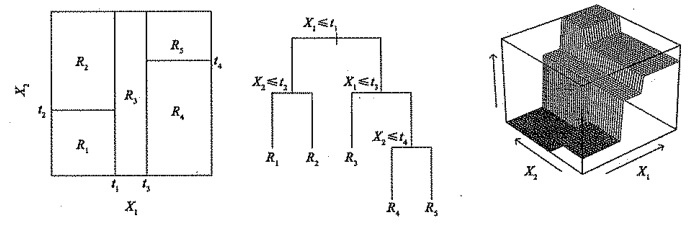回归树的剪枝
上述方法生成的回归树会在训练集中取得良好的预测效果，却很有可能造成数据的过拟合，导致在测试集上效果不佳。原因在于这种方法产生的树可能过于复杂。一棵分裂点更少、规模更小（区域

{

R

1

,

R

2

,

R

3

,

⋯

,

R

J

}

\{ R_1, R_2, R_3, \cdots, R_J\}

的个数更少）的树会有更小的方差和更好的可解释性（以增加微小偏差为代价）。针对上述问题，一种可能的解决办法是：仅当分裂使残差平方和

R

S

S

的减小量超过某阈值时，才分裂树结点。这种策略能生成较小的树，但可能产生过于短视的问题，一些起初看来不值得的分裂却可能之后产生非常好的分裂。也就是说在下一步中，

R

S

S

会大幅减小。
因此，更好的策略是生成一棵很大的树

T

0

T_0

α

\alpha

标记的一系列子树。每一个

α

\alpha

的取值对应一棵子树

T

∈

T

0

T \in T_0

，当

α

\alpha

一定时，其对应的子树使下式最小：

∑

m

=

1

∣

T

∣

∑

x

i

∈

R

m

(

y

i

−

y

^

R

m

)

2

+

α

∣

T

∣

\sum_{m=1}^{|T|} \sum_{x_i \in R_m}(y_i - \hat{y}_{R_m})^2 + \alpha |T|

这里的

∣

T

∣

|T|

表示树

T

T

的结点数，

R

m

R_m

是第

m

m

个终端结点对应的矩形（预测向量空间的一个子集），

y

^

R

m

\hat{y}_{R_m}

是与

R

m

R_m

对应的预测值，也就是

R

m

R_m

中训练集的平均值。调整系数

α

\alpha

在子树的复杂性和与训练数据的契合度之间控制权衡。当

α

=

0

\alpha = 0

时，子树

T

T

等于原树

T

0

T_0

，因为此时上式只衡量了训练误差。而当

α

\alpha

增大时，终端结点数多的树将为它的复杂付出代价，所以使上式取到最小值的子树会变得更小。
当

α

\alpha

从0开始逐渐增加时，树枝以一种嵌套的、可预测的模式被修剪，因此获得与

α

\alpha

对应的所有子树序列是很容易的。可以用交又验证或验证集确定

α

\alpha

，然后在整个数据集中找到与之对应的子树：

回归决策树算法 1.利用递归二叉分裂在训练集中生成一额大树，只有当终端结点包含的观测值个数低于某个最小值时才停止。 2.对大树进行代价复杂性剪枝，得到一系列最优子树，子树是

α

\alpha

的函数。 3.利用

K

K

折交叉验诞选择

α

\alpha

。具体做法是将训练集分为

K

K

折。对所有

k

=

1

,

2

,

3

,

⋯

,

K

k=1, 2, 3, \cdots, K

，对训练集上所有不属于第

k

k

折的数据重复第(1)步~第(2)步得到与

α

\alpha

对应的子树，并求出上述子树在第

k

k

折上的均方预测误差。 4.每个

α

\alpha

会有相应的

K

K

个均方预测误差，对这

K

K

个值求平均，选出使平均误差最小的

α

\alpha

。 5.找出选定的

α

\alpha

在第(2)步中对应的子树。

决策树的基础即是上文所述的分类决策树与回归决策树，其预测准确性一般比回归和分类方法弱，但可以通过用集成学习方法组合大量决策树，显著提升树的预测效果，这些方法我将会在接下来的文章中继续阐述，欢迎关注学习讨论！
展开全文机器学习 决策树
• ## 回归树

千次阅读 2020-06-01 13:08:36
回归树，就是用树模型做回归问题，每一片叶子都输出一个预测值。预测值一般是叶子结点所含训练集输出的均值。 回归树的分支标准：标准方差（Standard Deviation）。 回归树使用某一特征将原集合分为多个子集，用...
回归树，就是用树模型做回归问题，每一片叶子都输出一个预测值。预测值一般是叶子结点所含训练集输出的均值。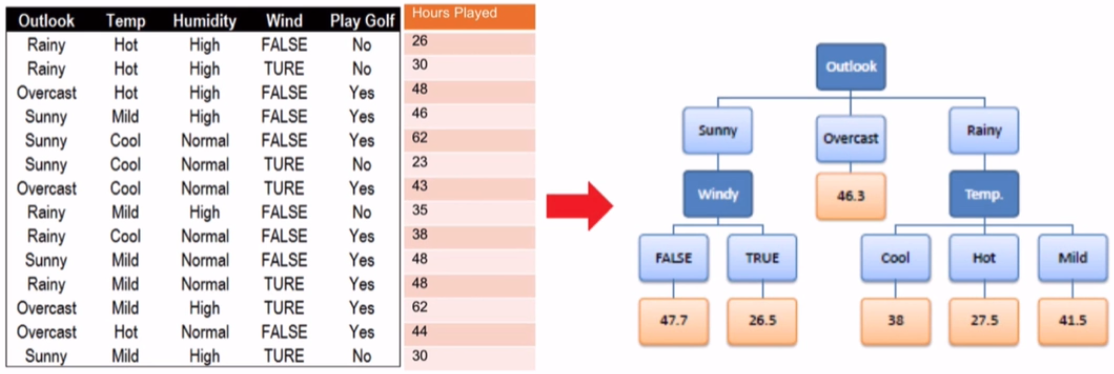回归树的分支标准：标准方差（Standard Deviation）。 回归树使用某一特征将原集合分为多个子集，用标准方差衡量子集中的元素是否接近，越小表示越接近。
首先计算根节点标准方差：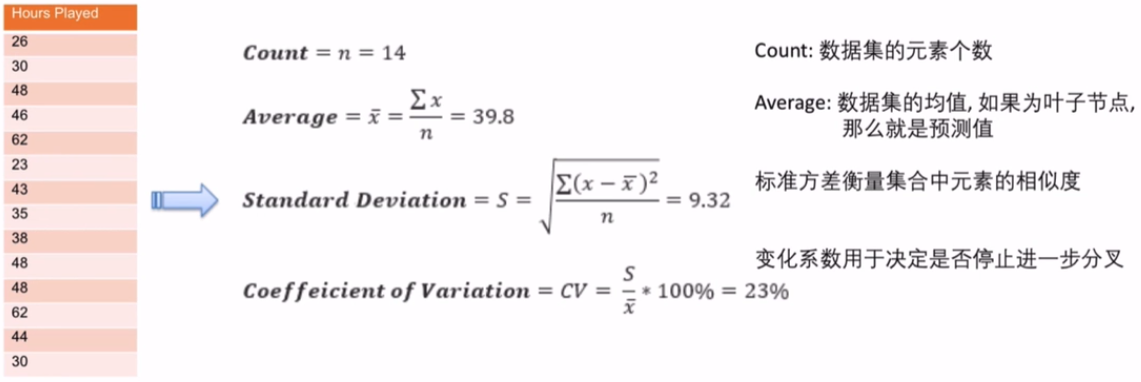使用标准方差来确定分支，以计算Outlook分支后的标准方差为例：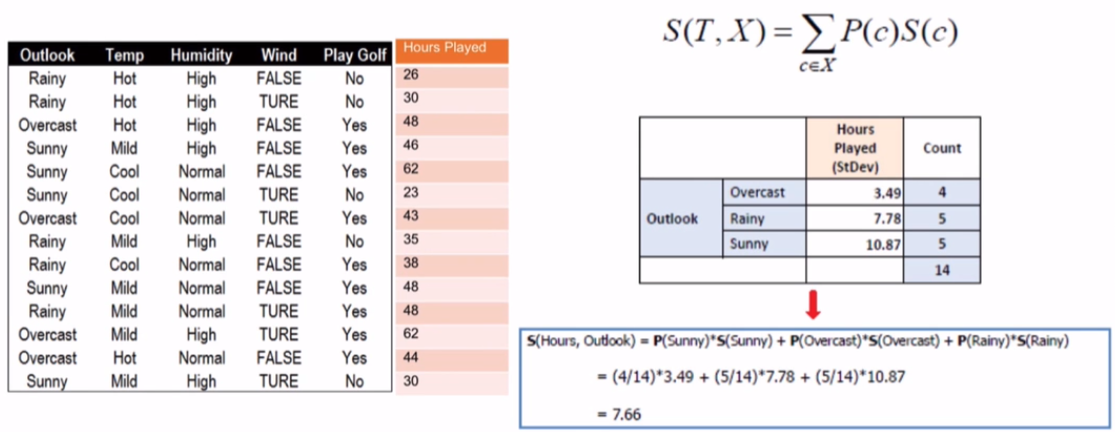同理可计算其他特征的标准差，并得到方差的减小值：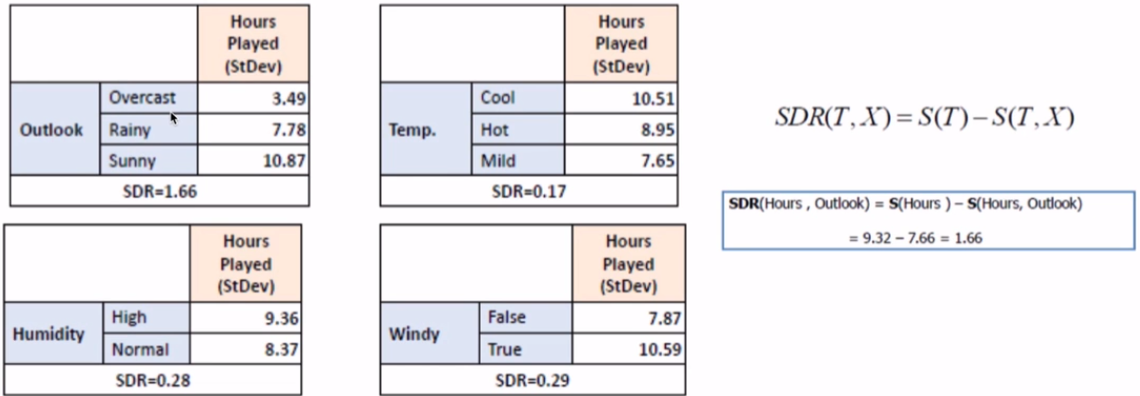标准差降低最多的特征是Outlook，利用其进行分支。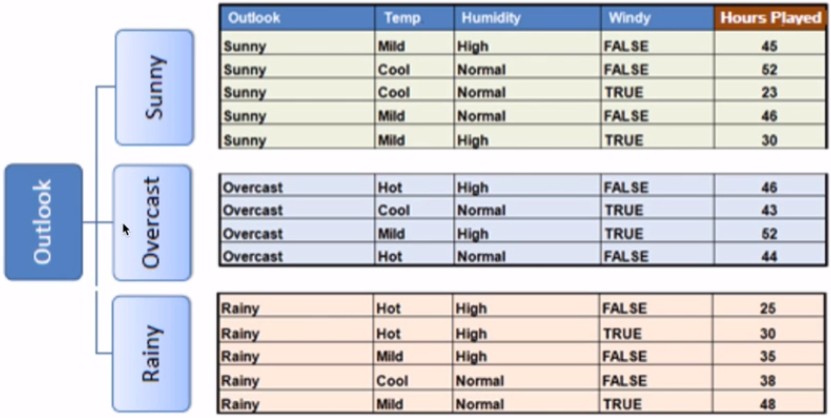接下来，重复这个过程，使用标准方差降低最多的特征进行分支。直到满足某个停止条件，如：
当某个分支的变化系数小于某个值（10%）当前节点包含的元素个数小于某个值（3）
使用Outlook分支以后，值为Overcast的分支的变化系数太小（8%），小于我们设置的最小值（10%），停止继续在Overcast对应的分支上继续分支，生成一个叶子结点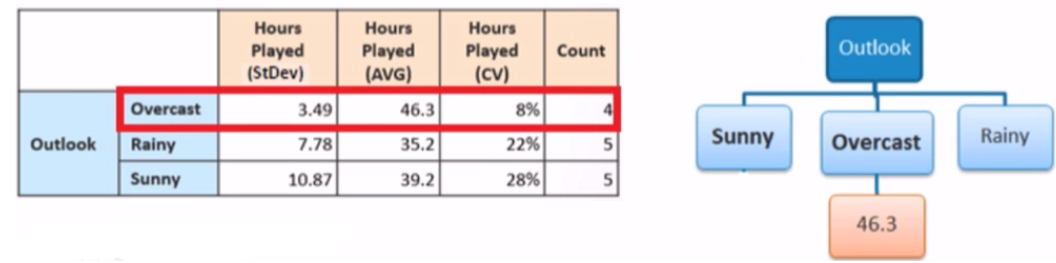再来看Sunny分支和Rainy分支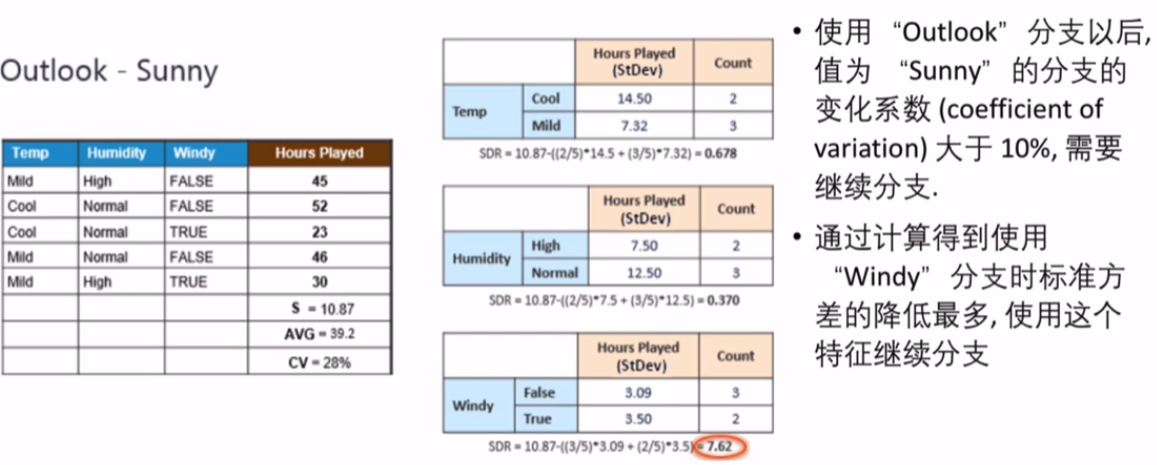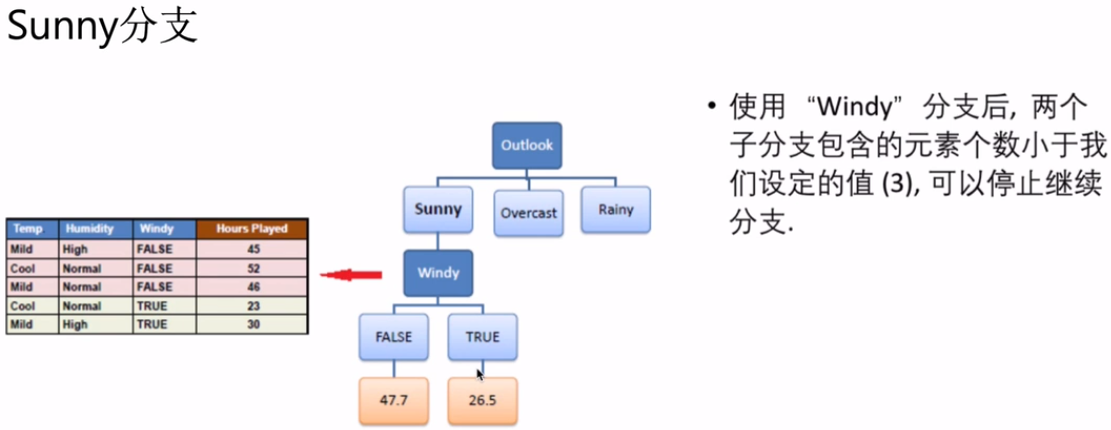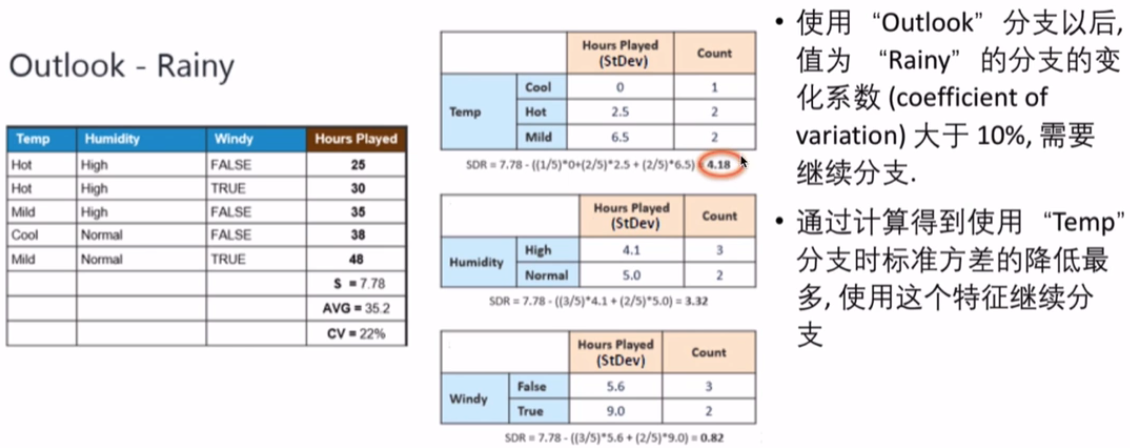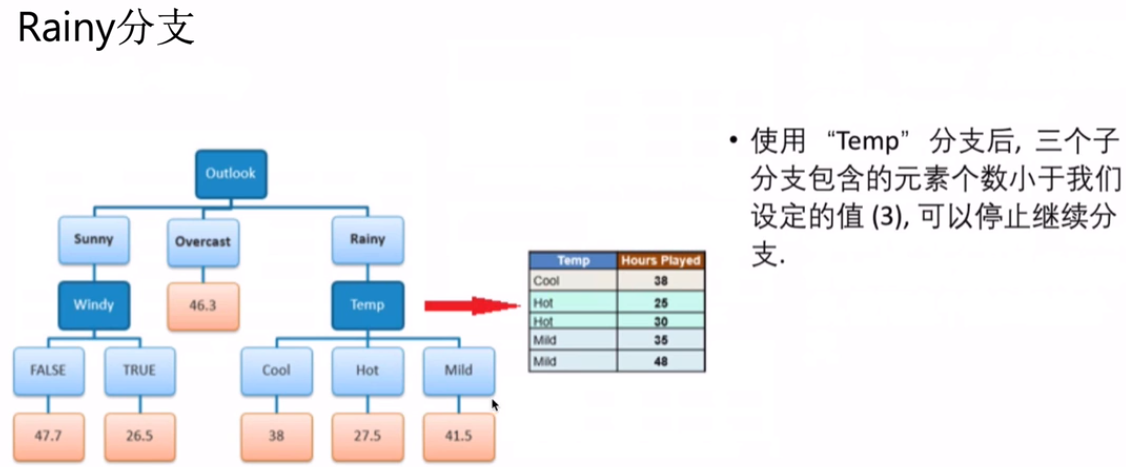最终的创建回归树结构如下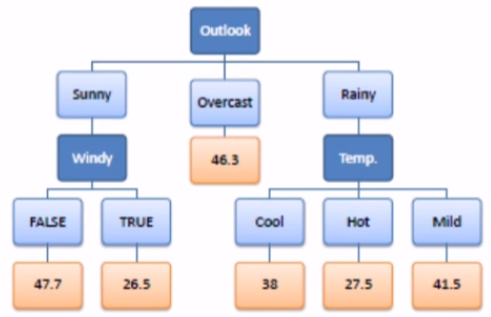展开全文• ## Regression Tree 回归树

万次阅读 多人点赞 2018-02-09 18:22:25
当前，最火的两类算法莫过于神经网络算法（CNN、RNN、LSTM等）与形算法（随机森林、GBDT、XGBoost等），形算法的基础就是决策。决策因其易理解、易构建、速度快的特性，被广泛应用于统计学、数据挖掘、机器...
1. 引言
AI时代，机器学习算法成为了研究、应用的热点。当前，最火的两类算法莫过于神经网络算法（CNN、RNN、LSTM等）与树形算法（随机森林、GBDT、XGBoost等），树形算法的基础就是决策树。决策树因其易理解、易构建、速度快的特性，被广泛应用于统计学、数据挖掘、机器学习领域。因此，对决策树的学习，是机器学习之路必不可少的一步。
根据处理数据类型的不同，决策树又分为两类：分类决策树与回归决策树，前者可用于处理离散型数据，后者可用于处理连续型数据，下面的英文引用自维基百科。

Classification tree analysis is when the predicted outcome is the class to which the data belongs.
Regression tree analysis is when the predicted outcome can be considered a real number (e.g. the price of a house, or a patient’s length of stay in a hospital).

基于以上原因，本文简单介绍了回归树（Regression Tree），简单描述了CART算法，给出了回归树的算法描述，辅以简单实例以加深理解，最后是总结部分。
2. 回归树
决策树实际上是将空间用超平面进行划分的一种方法，每次分割的时候，都将当前的空间一分为二， 这样使得每一个叶子节点都是在空间中的一个不相交的区域，在进行决策的时候，会根据输入样本每一维feature的值，一步一步往下，最后使得样本落入N个区域中的一个（假设有N个叶子节点），如下图所示。三种比较常见的分类决策树分支划分方式包括：ID3, C4.5, CART。分类与回归树（classificationandregressiontree, CART)模型由Breiman等人在1984年提出，是应用广泛的决策树学习方法。CART同样由特征选择、树的生成及剪枝组成，既可以用于分类也可以用于回归。下面的英文引用自维基百科

The term Classification And Regression Tree (CART) analysis is an umbrella term used to refer to both of the above procedures, first introduced by Breiman et al. Trees used for regression and trees used for classification have some similarities - but also some differences, such as the procedure used to determine where to split.

下面介绍回归树。
2.1 原理概述
既然是决策树，那么必然会存在以下两个核心问题：如何选择划分点？如何决定叶节点的输出值？
一个回归树对应着输入空间（即特征空间）的一个划分以及在划分单元上的输出值。分类树中，我们采用信息论中的方法，通过计算选择最佳划分点。而在回归树中，采用的是启发式的方法。假如我们有n个特征，每个特征有

s

i

(

i

∈

(

1

,

n

)

)

s_i(i \in (1,n))

个取值，那我们遍历所有特征，尝试该特征所有取值，对空间进行划分，直到取到特征j的取值s，使得损失函数最小，这样就得到了一个划分点。描述该过程的公式如下：（如果看不到图请点击永久地址）

假设将输入空间划分为M个单元：

R

1

,

R

2

,

.

.

.

,

R

m

R_1,R_2,...,R_m

那么每个区域的输出值就是：

c

m

=

a

v

e

(

y

i

∣

x

i

∈

R

m

)

c_m=ave(y_i|x_i \in R_m)

也就是该区域内所有点y值的平均数。
举个例子。如下图所示，假如我们想要对楼内居民的年龄进行回归，将楼划分为3个区域

R

1

,

R

2

,

R

3

R_1, R_2, R_3

（红线），那么

R

1

R_1

的输出就是第一列四个居民年龄的平均值，

R

2

R_2

的输出就是第二列四个居民年龄的平均值，

R

3

R_3

的输出就是第三、四列八个居民年龄的平均值。2.2 算法描述
截图来自李航教授的统计学习方法2.3 一个简单实例
为了便于理解，下面举一个简单实例。训练数据见下表，目标是得到一棵最小二乘回归树。
x12345678910y5.565.75.916.46.87.058.98.799.05
选择最优切分变量j与最优切分点s
在本数据集中，只有一个变量，因此最优切分变量自然是x。
接下来我们考虑9个切分点

[

1.5

,

2.5

,

3.5

,

4.5

,

5.5

,

6.5

,

7.5

,

8.5

,

9.5

]

[1.5, 2.5, 3.5, 4.5, 5.5, 6.5, 7.5, 8.5, 9.5]

你可能会问，为什么会带小数点呢？类比于篮球比赛的博彩，倘若两队比分是96:95，而盘口是“让1分 A队胜B队”，那A队让1分之后，到底是A队赢还是B队赢了？所以我们经常可以看到“让0.5分 A队胜B队”这样的盘口。在这个实例中，也是这个道理。
损失函数定义为平方损失函数

L

o

s

s

(

y

,

f

(

x

)

)

=

(

f

(

x

)

−

y

)

2

Loss(y, f(x))=(f(x)-y)^2

，将上述9个切分点一依此代入下面的公式，其中

c

m

=

a

v

e

(

y

i

∣

x

i

∈

R

m

)

c_m=ave(y_i|x_i \in R_m)

（如果看不到图请点击永久地址）

例如，取

s

=

1.5

s=1.5

。此时

R

1

=

{

1

}

,

R

2

=

{

2

,

3

,

4

,

5

,

6

,

7

,

8

,

9

,

10

}

R_1=\{1\} , R_2=\{2,3,4,5,6,7,8,9,10\}

，这两个区域的输出值分别为：

c

1

=

5.56

,

c

2

=

1

9

(

5.7

+

5.91

+

6.4

+

6.8

+

7.05

+

8.9

+

8.7

+

9

+

9.05

)

=

7.50

c_1=5.56, c_2= \frac{1}{9}(5.7+5.91+6.4+6.8+7.05+8.9+8.7+9+9.05)=7.50

。得到下表：
s1.52.53.54.55.56.57.58.59.5

c

1

c_1

5.565.635.725.896.076.246.626.887.11

c

2

c_2

7.57.737.998.258.548.918.929.039.05
把$c_1, c_2$的值代入到上式，如：$m(1.5)=0+15.72=15.72$。同理，可获得下表：

s1.52.53.54.55.56.57.58.59.5m(s)15.7212.078.365.783.911.938.0111.7315.74
显然取 $s=6.5$时，$m(s)$最小。因此，第一个划分变量$j=x, s=6.5$

用选定的(j,s)划分区域，并决定输出值 两个区域分别是：

R

1

=

{

1

,

2

,

3

,

4

,

5

,

6

}

,

R

2

=

{

7

,

8

,

9

,

10

}

R_1=\{1,2,3,4,5,6\} , R_2=\{7,8,9,10\}

输出值

c

m

=

a

v

e

(

y

i

∣

x

i

∈

R

m

)

,

c

1

=

6.24

,

c

2

=

8.91

c_m=ave(y_i|x_i \in R_m),c_1=6.24,c_2=8.91

对两个子区域继续调用步骤1、步骤2 对

R

1

R_1

继续进行划分:
x123456y5.565.75.916.46.87.05取切分点

[

1.5

,

2.5

,

3.5

,

4.5

,

5.5

]

[1.5, 2.5, 3.5, 4.5, 5.5]

，则各区域的输出值

c

c

如下表
s1.52.53.54.55.5

c

1

c_1

5.565.635.725.896.07

c

2

c_2

6.376.546.756.937.05计算m(s)：
s1.52.53.54.55.5m(s)1.30870.7540.27710.43681.0644s=3.5时m(s)最小。 之后的过程不再赘述。  生成回归树 假设在生成3个区域之后停止划分，那么最终生成的回归树形式如下：

T

=

{

5.72

x

≤

3.5

6.75

3.5

⩽

x

≤

6.5

8.91

6.5

<

x

T=\left\{\begin{matrix}5.72 & x\leq 3.5\\ 6.75 &3.5\leqslant x\leq 6.5\\ 8.91 & 6.5<x\end{matrix}\right.

2.4 回归树VS线性回归
不多说了，直接看图甩代码3. 总结
实际上，回归树总体流程类似于分类树，分枝时穷举每一个特征的每一个阈值，来寻找最优切分特征j和最优切分点s，衡量的方法是平方误差最小化。分枝直到达到预设的终止条件(如叶子个数上限)就停止。
当然，处理具体问题时，单一的回归树肯定是不够用的。可以利用集成学习中的boosting框架，对回归树进行改良升级，得到的新模型就是提升树（Boosting Decision Tree），在进一步，可以得到梯度提升树（Gradient Boosting Decision Tree，GBDT），再进一步可以升级到XGBoost。

作者邮箱： mr.yxj@foxmail.com
转载请告知作者，感谢!
展开全文机器学习 算法 Python 人工智能 大数据
• 面板回归树 介绍 该代码使用每个叶子中的固定效果面板回归模型来实现决策树。 这种方法将经典回归树扩展到面板结构化数据，并允许在叶子中拟合更复杂的模型。 该树的生长方式与经典回归树相同，即通过最小化方差。 ...R
• 决策树之CART（分类回归树）详解，具体内容如下 1、CART分类回归树简介   CART分类回归树是一种典型的二叉决策树，可以处理连续型变量和离散型变量。如果待预测分类是离散型数据，则CART生成分类决策树；如果待...
• CART（分类回归树）之回归树总结与树剪枝总结 CART（Classification And Regression Trees，分类回归树）与分类算法中决策树ID3算法最大的不同点在于，决策树是一种贪心算法，其要在给定时间内做出最佳选择，但并不...
    CART（分类回归树）之回归树总结与树剪枝总结
CART（Classification And Regression Trees，分类回归树）与分类算法中决策树ID3算法最大的不同点在于，决策树是一种贪心算法，其要在给定时间内做出最佳选择，但并不关心能否到达全局最优，不能直接处理连续型特征，且决策树特征切分份数由该特征值份数决定；分类回归树采用二元切分法（符合阈值条件进入左子树，否则进入右子树）来处理连续型变量，其不仅可以用于分类，还可以用于回归。
通过遍历数据集中所有特征及各个特征的所有特征值，根据每个特征值进行二元切分，计算切分后误差（一般取叶子结点数值的方差和），取最小误差对应取值作为切分阈值，进行迭代，得到各个树节点对应切分特征与切分阈值。如果最后特征值数目为1或新切分后误差与当前误差比较减小不大或切分出数据集很小，则退出切分，返回叶子节点或返回切分特征或切分特征值。
剪枝（pruning）
剪枝处理是为了防止树过拟合，其又有预剪枝和后剪枝之分。
预剪枝操作的一种为之前所属的根据切分数据集大小限制和新切分后误差与当前误差比较容限来提前终止切分。
后剪枝操作是通过比较叶子节点合并前后误差大小来进行，如果叶子结点合并后误差小于合并前，则进行剪枝，两个叶子节点合并，否则不合并（不进行剪枝操作）。
以后有时间补例程。`
展开全文• ## 决策树与回归树区别到底在哪

万次阅读 多人点赞 2019-03-19 21:01:03
可能很多人不用回归树做任务的时候很少去管回归树，以至于有时候也不知道它们的区别，但是还是有必要掌握，因为牛逼的树算法，比如GBDT，xgboost的单棵树可不是分类树，是回归树。 所谓分类树就是面向分类的，每个...决策树 机器学习
• 该档案库包含genfis4.m，该文件使用回归树算法生成Mamdani型和Sugeno型FIS，以从数据集中提取模糊规则信息。 它主要基于 Fuzzy Logic Toolbox，但需要修改 Toolbox 的模糊规则构建原理。 因此，一些原始的 m 文件...matlab
• CART，又名分类回归树，是在ID3的基础上进行优化的决策树，学习CART记住一下关键点： (1) CART既能是分类树，又能是回归树 (2) CART是分类树时，采用GINI指数作为节点分裂的依据；当CART是回归树时，采用样本的...
• ## 回归树预测

千次阅读 2017-07-05 17:01:53python
• 所谓的回归树模型其实就是用树形模型来解决回归问题，树模型当中最经典的自然还是决策树模型，它也是几乎所有树模型的基础。虽然基本结构都是使用决策树，但是根据预测方法的不同也可以分为两种。第一种，树上的叶子...
• 来源于知乎，本文就回归树的基本原理进行讲解，并手把手、肩并肩地带您实现这一算法。我们用人话而不是大段的数学公式来讲讲回归树是怎么一回事。如果预测某个连续变量的大小，最简单的模型之一就是用平均值。比如...
• 基于模糊c回归聚类和层次建模概念的协同组合，提出了一种新的回归树识别工具。 在特殊情况下 (c = 2)，模糊 c 回归聚类可用于识别铰链超平面模型。 所提出的方法通过划分一个局部线性模型的操作区域来递归地识别...matlab
• 如果将决策树应用于回归，则它可能指的是回归树。 决策树也称为回归树。 在本文中，我们专注于线性回归和回归树的概念。 来自UCI（机器学习存储库）的数据集用于这项研究工作。 该研究的目的是区分从线性回归和回归...
• 文章目录分类树与回归树回归树原理介绍最小二乘回归树生成算法CART算法Python代码节点类回归树类简单的例子Python库 分类树与回归树 分类树用于分类问题。分类决策树在选取划分点，用信息熵、信息增益、或者信息增益...
• 回归树 这个项目是训练一个回归树。 该项目基于 Jama 库。Java
• ## 决策树-CART回归树

千次阅读 2018-09-16 18:26:34
CART，又名分类回归树，是在ID3的基础上进行优化的决策树，学习CART记住以下几个关键点： （1）CART既能是分类树，又能是分类树； （2）当CART是分类树时，采用GINI值作为节点分裂的依据；当CART是回归树时，采用...
• 关于CART分类回归树的详细介绍以及它的应用实例。对于想要了解或学习CART分类回归树的同学，会有所帮助。
•研究论文
• 决策回归树主要用CART算法来实现。本资料包括有决策回归树的python实现以及相应的数据集，可以自动生成相应的决策树图。机器学习
• matlab开发-增强的二元回归树。增强二叉回归树是一种处理向量目标的强大的回归方法。
• Classification And Regression Tree(CART)是一种很重要的机器学习算法，既可以用于创建分类树（Classification Tree），也可以用于创建回归树（Regression Tree），本文介绍了CART用于离散标签分类决策和连续特征...算法 机器学习
• 下面介绍的回归树和另一篇文章介绍的分类树，都属于决策树范畴。分类树的模型是每个非叶子节点都是一个分类特征，按照该分类特征的不同取值，将数据集分为多少个子集；并且分类树模型我们要找的是测试数据集的最终...机器学习
• 这里我们使用CART算法来构建回归树和模型树。ID3算法是每次选取当前最佳的特征来分割数据，并按照该特征的所有可能取值来区分。比如，如果一个特征有4种取值，那么数据将被切分成4份。很明显，该算法不适用于标签值...python 模型树
• 分类回归树与随机森林分类回归树与随机森林分类回归树与随机森林...